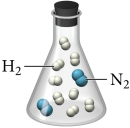# Problem: Nitrogen and hydrogen gas react to form ammonia according to the reaction:N2(g) + 3H2(g) → 2NH3(g)What is the limiting reactant?

###### FREE Expert Solution
N2(g) + 3 H2(g) → 2 NH3(g)In the container:   2 moles N2 and 8 moles H2

Calculate the amount of NH3 produced from each reactant and determine the limiting reactant:

82% (5 ratings)###### Problem Details
Nitrogen and hydrogen gas react to form ammonia according to the reaction:

N2(g) + 3H2(g) → 2NH3(g)What is the limiting reactant?

Frequently Asked Questions

What scientific concept do you need to know in order to solve this problem?

Our tutors have indicated that to solve this problem you will need to apply the Limiting Reagent concept. You can view video lessons to learn Limiting Reagent. Or if you need more Limiting Reagent practice, you can also practice Limiting Reagent practice problems.